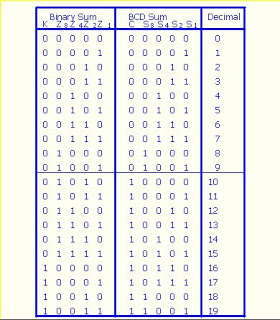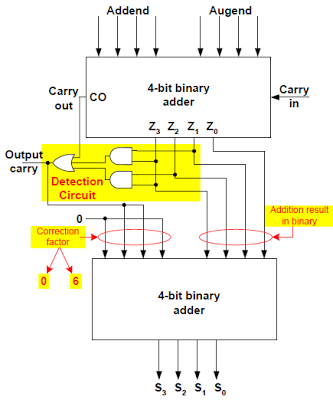## 8240819346

##### EMAIL:
###### info@mcatutorials.com
Enroll now!
 Name Cources BTECH MCA MSC BCA BSC Others -- Choose Your Subject -- Computer Organization C Language Data Structure Digital Electronics Object Oriented Programming Discrete Mathematics Graph Theory Operating Systems Software Engineering Computer Graphics Database Management System Operation Research Computer Networking Image Processing Internet Technologies Micro Processor Artificial Intelligence Soft Computing E-Commerce & ERP C ++ Language C Programming C Data Structure Using C, C ++ Sql & PL/Sql Visual Basic Unix and Shell programming Java J2EE Oracle 9i 8085 Programming Android Application Development i-phone Application Development JAVA Swing based applications PHP, MySql, Ajax ASP.net Visual Basic Client Server Applications Email PhoneBCD is a class of encoding in which each decimal digit is represented by some fixed number of bits .Usually 4 or 8 bits are used .

 Decimal BCD 0 0000 1 0001 2 0010 3 0011 4 0100 5 0101 6 0110 7 0111 8 1000 9 1001

up to 9 the bcd representation is same as the decimal representation and after the 9 <

 Decimal BCD 10 0001 0000 11 0001 0001 12 0001 0010 13 0001 0011 14 0001 0100 15 0001 0101 16 0001 0110 17 0001 1000 18 0001 1000 19 0001 1001

the first 4 digits in BCD representation is used to show the first digit in decimal and next four digits in BCD are used to represent next digit in decimal .

## TRUTH TABLE## WHY only 4 BIT ADDER CAN NOT BE USED ?

when we provide two 4 bits BCD number to the 4 bit adders , the output exceeds the BCD range , or called BCD representation . WE WANT TO OUTPUT ALSO IN BCD . but when we directly take the output of the 4 bit adder then it will be a invalid representation . Therefore we need some mechanism through which we can change the output of the 4 bit adder into a valid BCD representation .

if you carry fully see , that the up to when the sum is 9 , the BCD representation is same as the binary representation .

when the sum exceed the 9 , the following condition occur :-
1) either the carry bit is 1
2) the sum is 1010.
3) or the sum is 1100.

for the numbers which does not satisfy the condition of BCD , 6 is added . see in the table , when the sum is 01010 the bcd representation is obtained by adding 6 ., so the representation in BCD is 1 0000.
the condition when 6 is to be added is
F = CO + Z3 Z2 + Z3 Z1

this can be easily obtained by K - map .
where CO is the output carry . and the z3 is the 4 bit , z2 the bit 3 and similarly z1 bit 2